#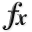N-D Test Functions A¶

class go_benchmark.Ackley(dimensions=2)

Ackley test objective function.

This class defines the Ackley global optimization problem. This is a multimodal minimization problem defined as follows: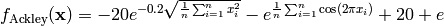Here,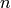represents the number of dimensions andfor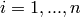.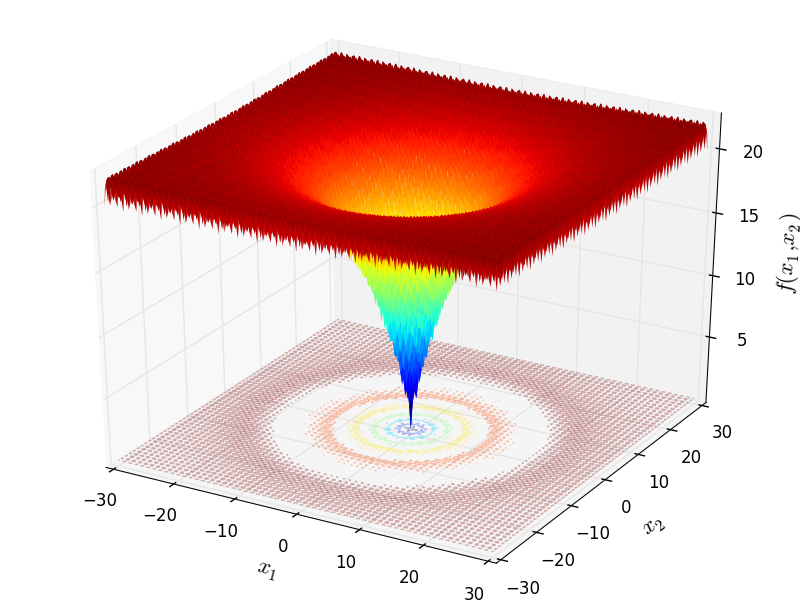Two-dimensional Ackley function

Global optimum: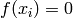for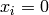forThis class defines the Adjiman global optimization problem. This is a multimodal minimization problem defined as follows: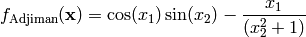Here,represents the number of dimensions and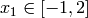and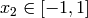.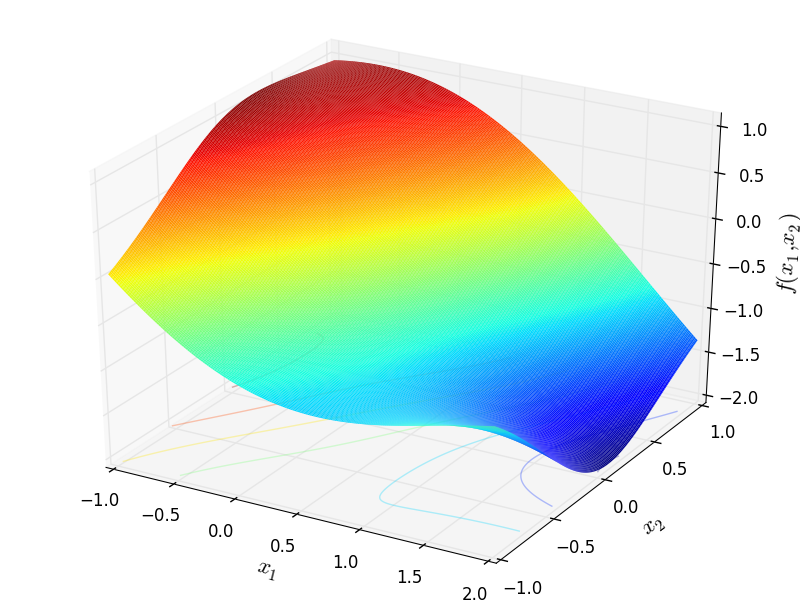Global optimum: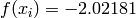for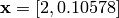class go_benchmark.Alpine01(dimensions=2)

Alpine 1 test objective function.

This class defines the Alpine 1 global optimization problem. This is a multimodal minimization problem defined as follows: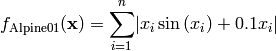Here,represents the number of dimensions andfor.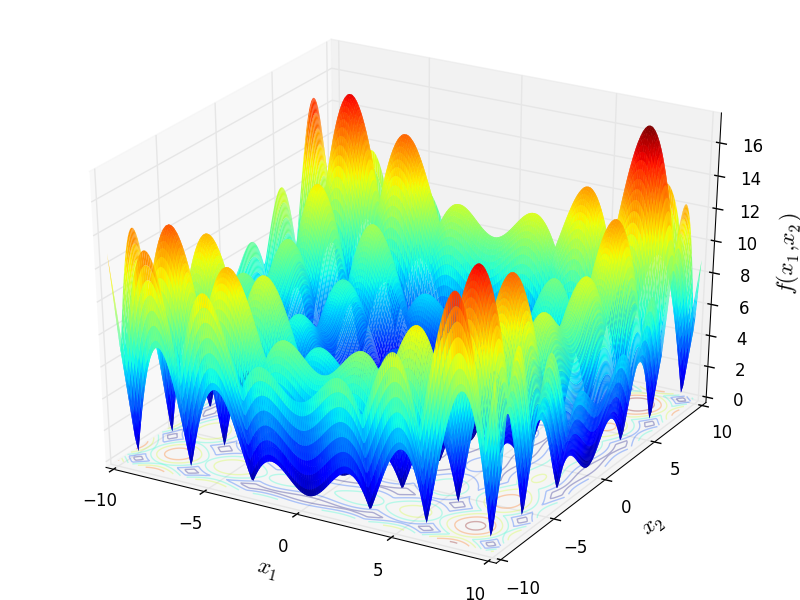Two-dimensional Alpine 1 function

Global optimum:forforclass go_benchmark.Alpine02(dimensions=2)

Alpine 2 test objective function.

This class defines the Alpine 2 global optimization problem. This is a multimodal minimization problem defined as follows:Here,represents the number of dimensions and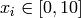for.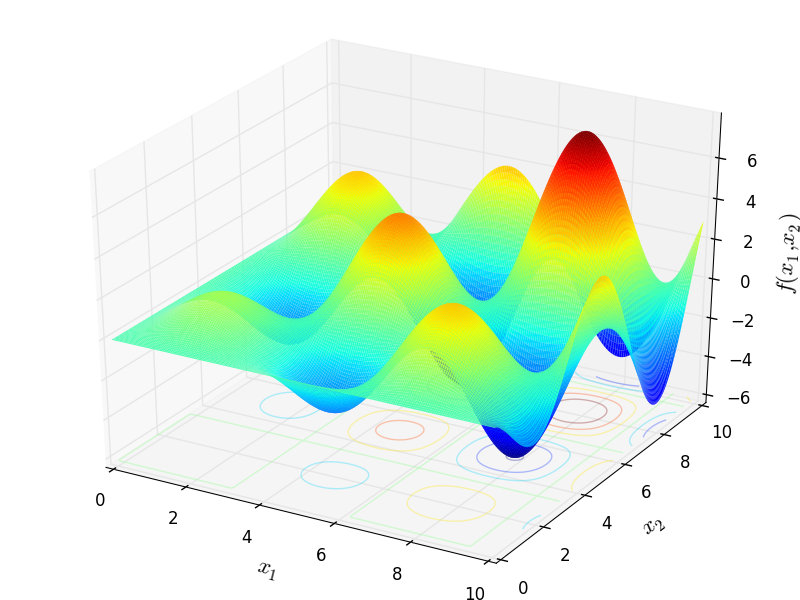Two-dimensional Alpine 2 function

Global optimum:for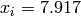forclass go_benchmark.AMGM(dimensions=2)

AMGM test objective function.

This class defines the Arithmetic Mean - Geometric Mean Equality global optimization problem. This is a multimodal minimization problem defined as follows:Here,represents the number of dimensions andfor.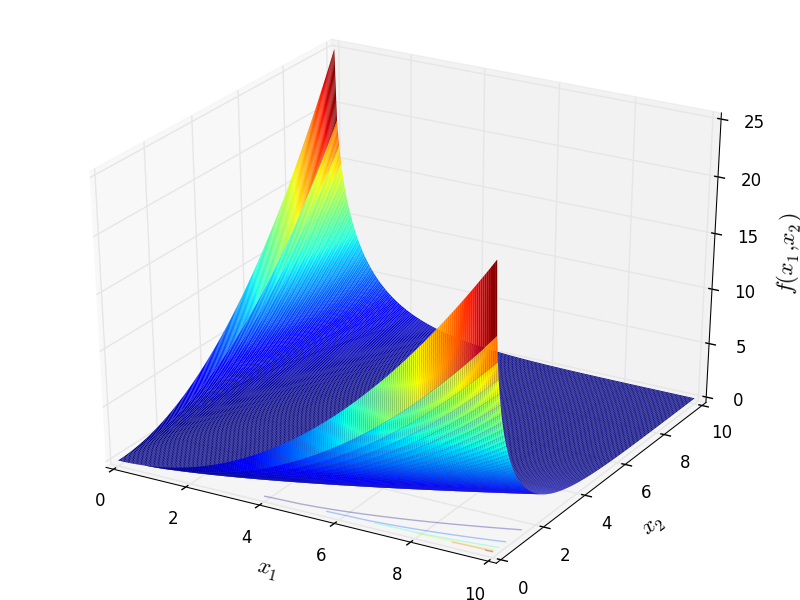Two-dimensional Arithmetic Mean - Geometric Mean Equality function

Global optimum:for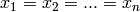for#### Previous topic

Test Functions Index

#### Next topic

N-D Test Functions B